# ISEE Middle Level Quantitative : How to use a Venn Diagram

## Example Questions

### Example Question #1 : How To Use A Venn Diagram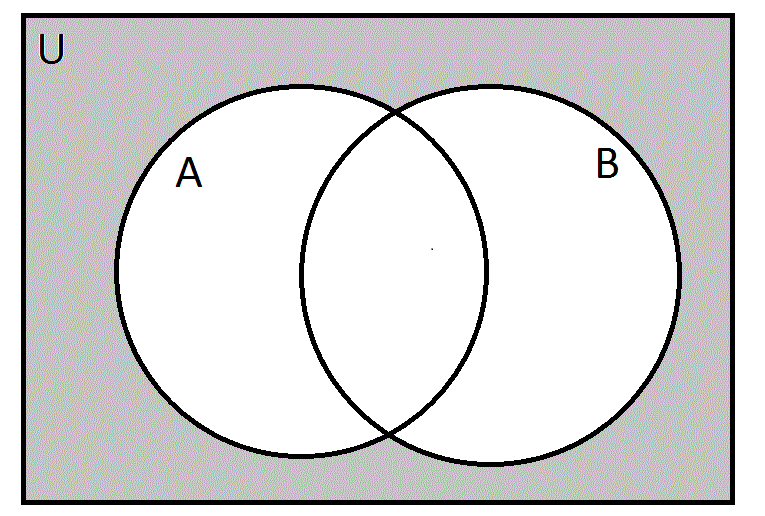Define:

Universal set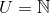, the set of all natural numbers.= {|is a multiple of 3}= {|is a multiple of 5}

If all of the natural numbers were to be placed in their appropriate places in the above Venn diagram, which of the following would be placed in the gray region?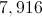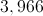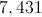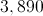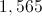Explanation:

The gray region is the region outside bothandand is therefore the set of natural numbers that are multiples of neither 3 nor 5.

1,565 and 3,890 can be eliminated as choices as each is divisible by 5: the former ends in 5 and the latter ends in 0.

7,431 and 3,966 can be eliminated as each is divisible by 3: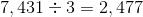and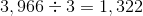7,916 is not divisible by 5 as it ends in 6; it is not divisible by 3 as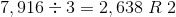. Therefore, it is the correct choice.

### Example Question #2 : How To Use A Venn DiagramDefine:

Universal set, the set of all natural numbers.= {|is a multiple of 3}= {|is a multiple of 5}

If all of the natural numbers were to be placed in their appropriate places in the above Venn diagram, which of the following would be placed in the gray region?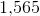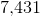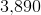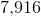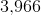Explanation:

The gray region is the region outside bothandand is therefore the set of natural numbers that are multiples of neither 3 nor 5.

1,565 and 3,890 can be eliminated as choices as each is divisible by 5: the former ends in 5 and the latter ends in 0.

7,431 and 3,966 can be eliminated as each is divisible by 3:and7,916 is not divisible by 5 as it ends in 6; it is not divisible by 3 as. Therefore, it is the correct choice.